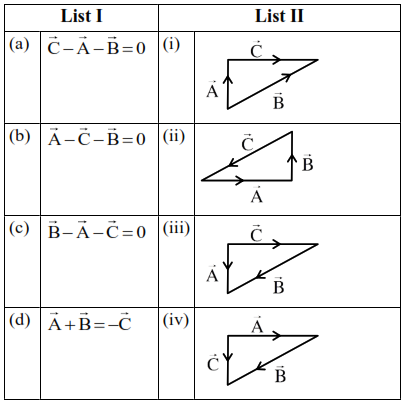# Match List I with List II.

Question:

Match List I with List II.Choose the correct answer from the options given below :

1. (a) $\rightarrow$ (iv), (b) $\rightarrow$ (i) , (c) $\rightarrow$ (iii), (d) $\rightarrow$ (ii)

2. $(\mathrm{a}) \rightarrow(\mathrm{iv})$, (b) $\rightarrow($ iii $),(\mathrm{c}) \rightarrow(\mathrm{i}),(\mathrm{d}) \rightarrow(\mathrm{ii})$

3. (a) $\rightarrow$ (iii), (b) $\rightarrow$ (ii), (c) $\rightarrow$ (iv), (d) $\rightarrow$ (i)

4. $(\mathrm{a}) \rightarrow(\mathrm{i}),(\mathrm{b}) \rightarrow(\mathrm{iv})$, (c) $\rightarrow($ ii $),(\mathrm{d}) \rightarrow($ iii $)$

Correct Option: , 2

Solution:

(a) $\overrightarrow{\mathrm{C}}=\overrightarrow{\mathrm{A}}+\overrightarrow{\mathrm{B}}$

Option (iv)

(b) $\overrightarrow{\mathrm{A}}=\overrightarrow{\mathrm{B}}+\overrightarrow{\mathrm{C}}=\overrightarrow{\mathrm{C}}+\overrightarrow{\mathrm{B}}$

Option (iii)

(c) $\overrightarrow{\mathrm{B}}=\overrightarrow{\mathrm{A}}+\overrightarrow{\mathrm{C}}$

Option (i)

(d) $\overrightarrow{\mathrm{A}}+\overrightarrow{\mathrm{B}}+\overrightarrow{\mathrm{C}}=0$

Option (ii)